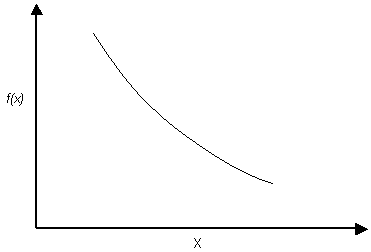# Exponential Distribution

The Exponential probability density function is available in RocTopple.

Exponential probability density functionNOTES:

• The range of values must always be positive for an Exponential distribution, therefore it should not be used for variables which may include negative values.
• The mean is always equal to the standard deviation for an Exponential distribution. This is a property of the Exponential distribution, and cannot be altered by the user.
• Like the Normal distribution, the Exponential distribution can be truncated by entering the desired minimum and maximum values (the basic Exponential distribution varies from zero to infinity).

The Exponential distribution is sometimes used to define events, such as the occurrence of earthquakes or rockbursts, or quantities such as the length of joints in a rock mass. Of the currently defined statistical variables in RocTopple, you may find an exponential distribution useful for modeling joint cohesion or water level, for example.